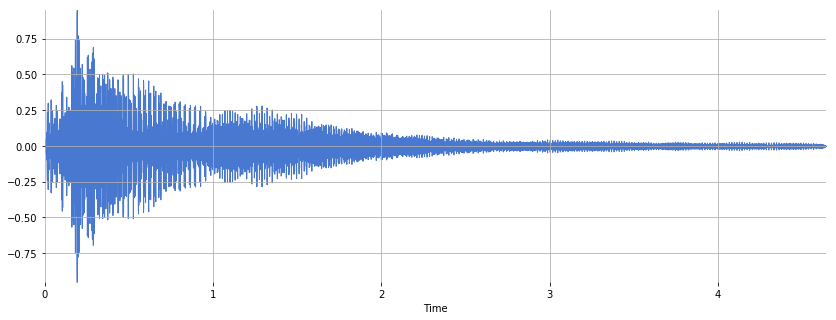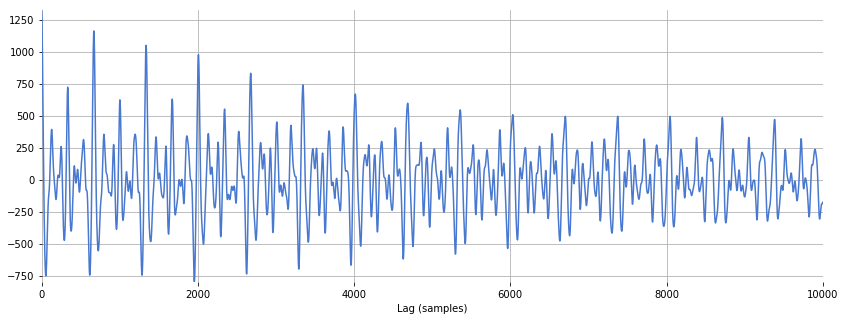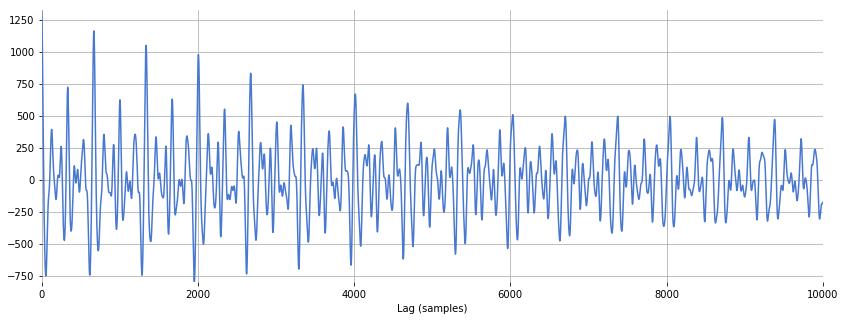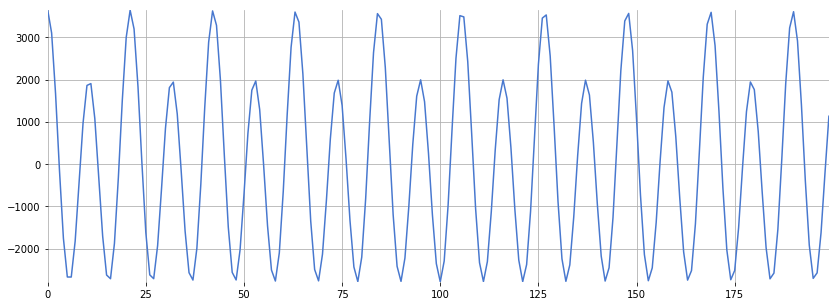In :
%matplotlib inline
import numpy, scipy, matplotlib.pyplot as plt, IPython.display as ipd
import librosa, librosa.display
import stanford_mir; stanford_mir.init()


# Autocorrelation¶

The autocorrelation of a signal describes the similarity of a signal against a time-shifted version of itself. For a signal $x$, the autocorrelation $r$ is:

$$r(k) = \sum_n x(n) x(n-k)$$

In this equation, $k$ is often called the lag parameter. $r(k)$ is maximized at $k = 0$ and is symmetric about $k$.

The autocorrelation is useful for finding repeated patterns in a signal. For example, at short lags, the autocorrelation can tell us something about the signal's fundamental frequency. For longer lags, the autocorrelation may tell us something about the tempo of a musical signal.

In :
x, sr = librosa.load('audio/c_strum.wav')
ipd.Audio(x, rate=sr)

Out:
In :
plt.figure(figsize=(14, 5))
librosa.display.waveplot(x, sr)

Out:
<matplotlib.collections.PolyCollection at 0x1172659e8>### numpy.correlate¶

There are two ways we can compute the autocorrelation in Python. The first method is numpy.correlate:

In :
# Because the autocorrelation produces a symmetric signal, we only care about the "right half".
r = numpy.correlate(x, x, mode='full')[len(x)-1:]
print(x.shape, r.shape)

(102400,) (102400,)


Plot the autocorrelation:

In :
plt.figure(figsize=(14, 5))
plt.plot(r[:10000])
plt.xlabel('Lag (samples)')
plt.xlim(0, 10000)

Out:
(0, 10000)### librosa.autocorrelate¶

The second method is librosa.autocorrelate:

In :
r = librosa.autocorrelate(x, max_size=10000)
print(r.shape)

(10000,)

In :
plt.figure(figsize=(14, 5))
plt.plot(r)
plt.xlabel('Lag (samples)')
plt.xlim(0, 10000)

Out:
(0, 10000)librosa.autocorrelate conveniently only keeps one half of the autocorrelation function, since the autocorrelation is symmetric. Also, the max_size parameter prevents unnecessary calculations.

## Pitch Estimation¶

The autocorrelation is used to find repeated patterns within a signal. For musical signals, a repeated pattern can correspond to a pitch period. We can therefore use the autocorrelation function to estimate the pitch in a musical signal.

In :
x, sr = librosa.load('audio/oboe_c6.wav')
ipd.Audio(x, rate=sr)

Out:

Compute and plot the autocorrelation:

In :
r = librosa.autocorrelate(x, max_size=5000)
plt.figure(figsize=(14, 5))
plt.plot(r[:200])

Out:
[<matplotlib.lines.Line2D at 0x116f41240>]The autocorrelation always has a maximum at zero, i.e. zero lag. We want to identify the maximum outside of the peak centered at zero. Therefore, we might choose only to search within a range of reasonable pitches:

In :
midi_hi = 120.0
midi_lo = 12.0
f_hi = librosa.midi_to_hz(midi_hi)
f_lo = librosa.midi_to_hz(midi_lo)
t_lo = sr/f_hi
t_hi = sr/f_lo

In :
print(f_lo, f_hi)
print(t_lo, t_hi)

16.351597831287414 8372.018089619156
2.633773573344376 1348.4920695523206


Set invalid pitch candidates to zero:

In :
r[:int(t_lo)] = 0
r[int(t_hi):] = 0

In :
plt.figure(figsize=(14, 5))
plt.plot(r[:1400])

Out:
[<matplotlib.lines.Line2D at 0x118225208>]